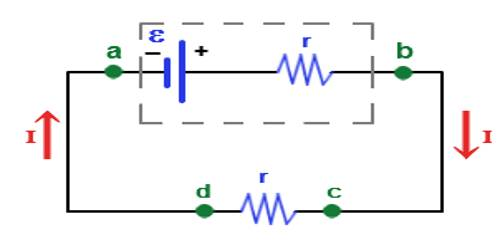Physics

# Electromotive ForceElectromotive force is a difference in potential that tends to give rise to an electric current. Energy is converted from one form to another in the generator or battery as the device does work on the electric charge being transferred within itself. It is the electric potential generated by either an electrochemical cell or a changing magnetic field.

Due to the presence of positive and negative charges in the two terminals of the cell, a potential difference appears between the terminals. The maximum potential difference that exists between the terminals of the cell, when it is disconnected from the external circuit, is called the electromotive force of the cell.

It is also denoted as e.m.f. is the energy provided by a cell or battery per coulomb of charge passing through it, it is measured in volts (V). It is equal to the potential difference between the terminals of the cell when no current is flowing.

ε = E / Q

• ε = electromotive force in volts, V
• E = energy in joules, J
• Q = charge in coulombs, C

Unit of emf: The unit of emf electromotive force is volt (V) or Joule/ Coulomb (J/C).